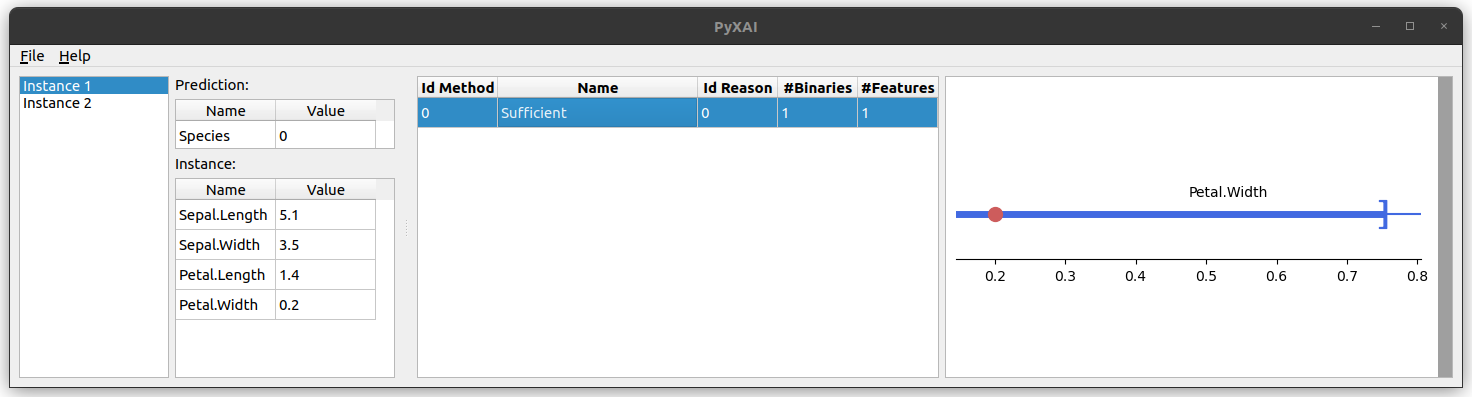PyXAI

### What is PyXAI ?

PyXAI (Python eXplainable AI) is a Python library (version 3.6 or later) allowing to bring formal explanations suited to (regression or classification) tree-based ML models (Decision Trees, Random Forests, Boosted Trees, ...). In contrast to many approaches to XAI (SHAP, LIME, ...), PyXAI algorithms are model-specific. Furthermore, PyXAI algorithms guarantee certain properties about the explanations generated, that can be of several types:

• Abductive explanations for an instance $X$ are intended to explain why $X$ has been classified in the way it has been classified by the ML model (thus, addressing the “Why?” question). In the regression case, abductive explanations for $X$ are intended to explain why the regression value of $X$ belongs to a given interval.
• Contrastive explanations for $X$ are intended to explain why $X$ has not been classified by the ML model as the user expected it (thus, addressing the “Why not?” question).

New features in version 1.0.0:

### What is the difference between PyXAI and other methods ?

The most popular approaches (SHAP, LIME, ...) to XAI are model-agnostic, but they do not offer any guarantees of rigor. A number of works have highlighted several misconceptions about such approaches to XAI (see the related papers). Contrastingly, PyXAI algorithms rely on logic-based, model-precise approaches for computing explanations. Although formal explainability has a number of drawbacks, particularly in terms of the computational complexity of logical reasoning needed to derive explanations, steady progress has been made since its inception.

### Which models can be explained with PyXAI ?

Models are the resulting objects of an experimental ML protocol through a chosen cross-validation method (for example, the result of a training phase on a classifier). Importantly, in PyXAI, there is a complete separation between the learning phase and the explaining phase: you produce/load/save models, and you find explanations for some instances given such models. Currently, with PyXAI, you can use methods to find explanations suited to different ML models for classification or regression tasks:

In addition to finding explanations, PyXAI also provides methods that perform operations (production, saving, loading) on models and instances. Currently, these methods are available for three ML libraries:

• Scikit-learn: a software machine learning library
• XGBoost: an optimized distributed gradient boosting library
• LightGBM: a gradient boosting framework that uses tree-based learning algorithms

It is also possible to leverage PyXAI to find explanations suited to models learned using other libraries.

### What does this website offer ?

In this website, you can find all what you need to know about PyXAI, with more than 10 Jupyter Notebooks, including:

• The installation guide and the quick start page
• The concepts used byt the PyXAI explainer module: Concepts
• Theories gathering pieces of knowledge about the dataset. PyXAI offers the possibility of encoding a theory when calculating explanations in order to avoid the computation of impossible explanations: Theories
• The PyXAI library offers the possibility to process user preferences (prefer some explanations to others or exclude some features): Preferences
• How to set a time limit? Time Limit
• How to compute explanations for classification tasks? Explaining Classification
• How to compute explanations for regression tasks? Explaining Regression
• How to use the PyXAI's Graphical User Interface (GUI) for visualizing explanations?

### How to use PyXAI ?

Here is an example (it comes from the Quick Start page):

#### PyXAI in action

from pyxai import Learning, Explainer

learner = Learning.Scikitlearn("tests/iris.csv", learner_type=Learning.CLASSIFICATION)
model = learner.evaluate(method=Learning.HOLD_OUT, output=Learning.DT)
instance, prediction = learner.get_instances(model, n=1, correct=True, predictions=)

explainer = Explainer.initialize(model, instance)
print("instance:", instance)
print("binary representation:", explainer.binary_representation)

sufficient_reason = explainer.sufficient_reason(n=1)
print("sufficient_reason:", sufficient_reason)
print("to_features:", explainer.to_features(sufficient_reason))

instance, prediction = learner.get_instances(model, n=1, correct=False)
explainer.set_instance(instance)
contrastive_reason = explainer.contrastive_reason()
print("contrastive reason", contrastive_reason)
print("to_features:", explainer.to_features(contrastive_reason, contrastive=True))

explainer.show()As illustrated by this example, with a few lines of code, PyXAI allows you to train a model, extract instances, and get explanations about the classifications made.